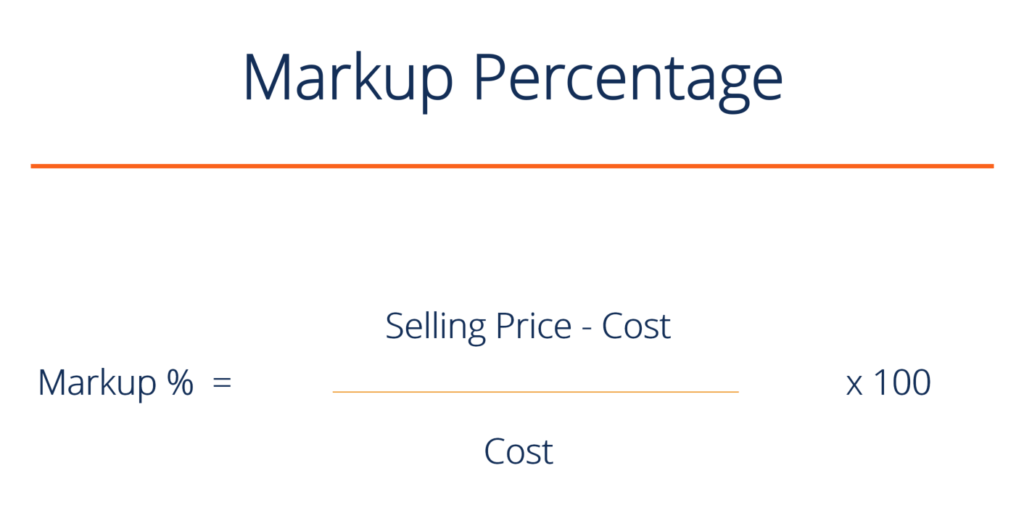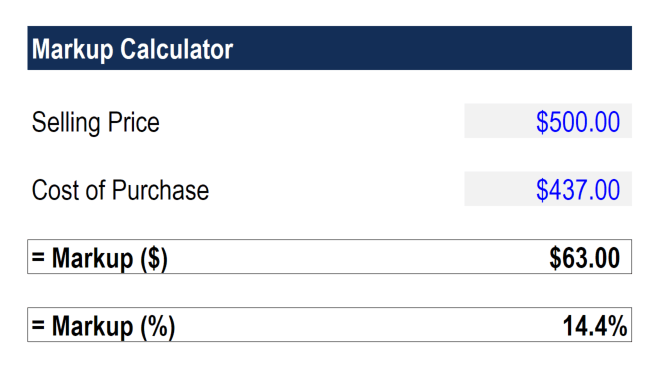# Markup Calculator & Formula

Calculate the markup on a good or service

## What is a markup percentage?

Markup percentage is a concept commonly used in managerial/cost accounting work and is equal to the difference between the selling price and cost of a good, divided by the cost of that good. This guide outlines the markup formula and also provides a markup calculator to download.

Markup percentages are especially useful in calculating how much to charge for the goods/services that a company provides its consumers. A markup percentage is a number used to determine the selling price of a product in relation to the cost of actually producing the product. The number expresses a percentage above and beyond the cost to calculate the selling price.  Markups are common in cost accounting, which focuses on reporting all relevant information to management to make internal decisions that better align with the company’s overall strategic goals.### Markup formula

The marketup formula is as follows:

Markup % = (selling price – cost) / cost x 100

Where the markup formula is dependent on,

Selling Price = the final sale price

Cost = the cost of the good

### Markup CalculatorInstructions how to use the markup calculator:

2. Enter the selling price of the product
3. Enter the cost of purchasing the product
4. View the markup in \$ and in %

### Example of a markup percentage

XYZ Company is a company that manufactures small gadgets. Its variable costs are \$50 per gadget and its fixed costs equal \$1,000. If the company implements a 30% markup rate, how much should each gadget sell for, assuming 500 gadgets are sold in total for the year?

Variable Costs per unit                       \$50

Fixed Cost per unit                                  2

Total Costs per unit                            \$52

Mark up percentage:                          30%

Selling price:                                     \$67.6

### Markup percentage vs gross margin

As an example, a markup of 40% for a product that cost \$100 to produce would sell for \$140. The Markup is different from gross margin because markup uses the cost of production as the basis for determining the selling price while gross margin is simply the difference between total revenue and the cost of goods sold. Markup percentages vary widely between different industries, product lines, and businesses. For instance, some products will have a markup of 5% while others will have a markup of 90%.

### Implications of markups

Using markup percentages is a simple and common way for companies to determine unit selling prices and meet profit goals. However, simply implementing a number ignores other factors that are pertinent to sales performance. For example, companies may increase the markup percentage to maximize their profit, which negates the idea of price elasticity.

Although it could be beneficial for companies, it is highly unlikely that sales will remain the same if markup percentages are increased, especially given the competitive market today.

A different way that companies could maximize their profit instead of altering markup percentages is to consider investments in machinery or PPE to increase their fixed costs and decrease variable costs if unit variable costs are too high. This would be effective if sales reach a certain level only.

Overall, markup percentages are just one way to determine selling price out of the numerous pricing strategies that use production costs as a basis.